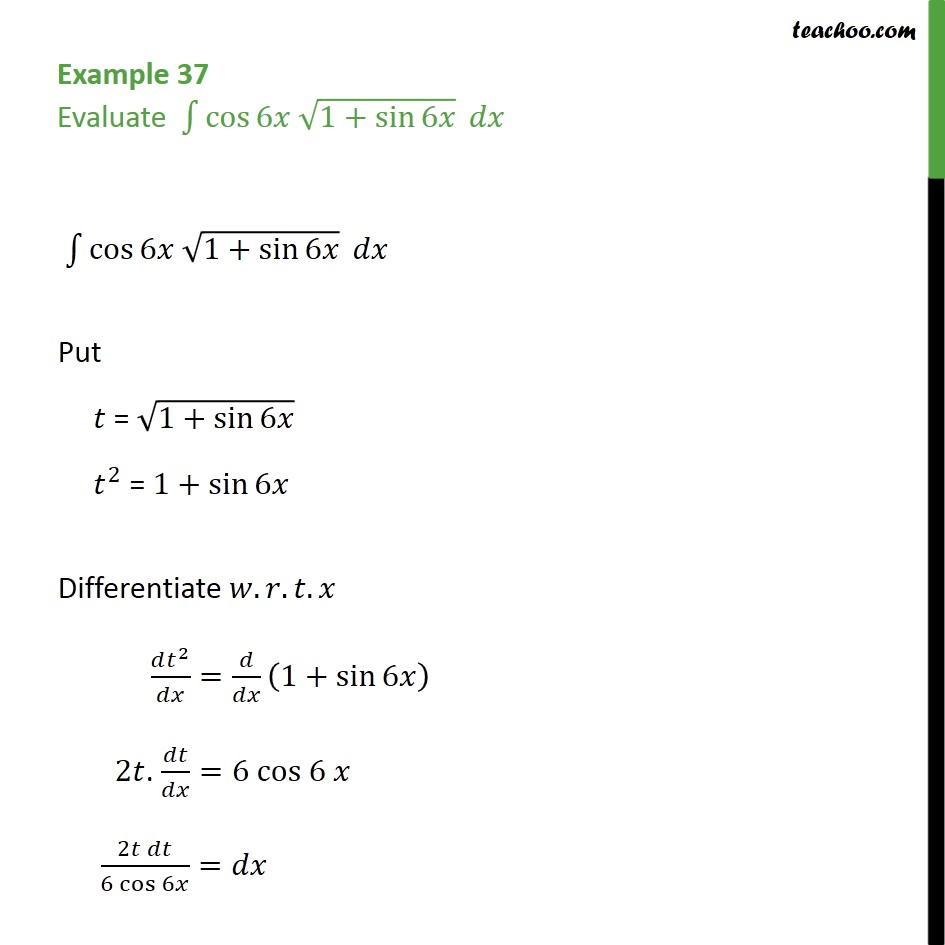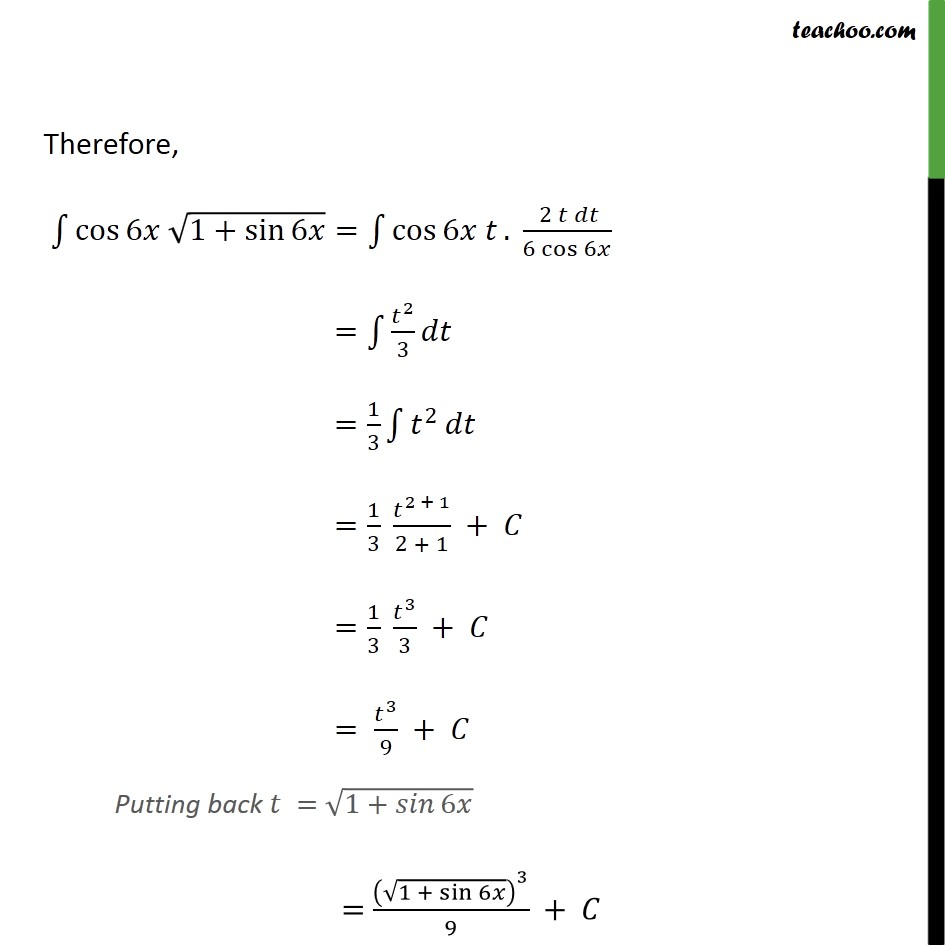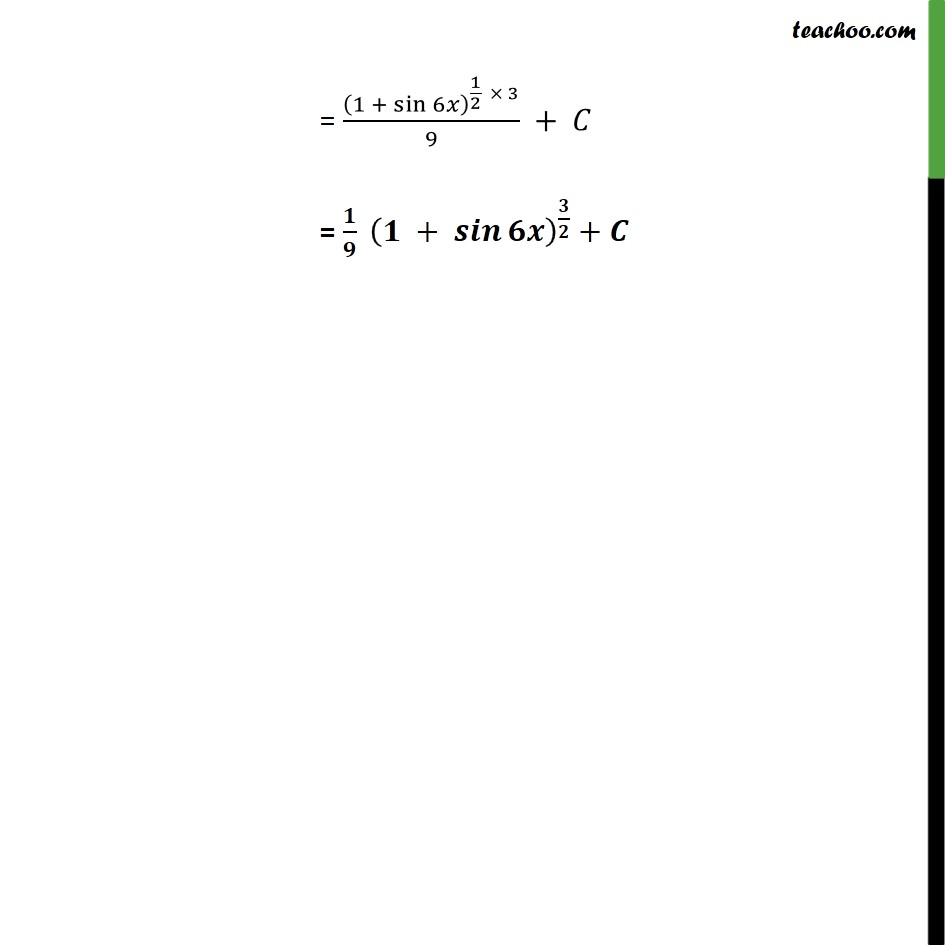1. Chapter 7 Class 12 Integrals (Term 2)
2. Concept wise
3. Integration by substitution - Trignometric - Normal

Transcript

Example 37 Evaluate cos 6 1+ sin 6 cos 6 1+ sin 6 Put = 1+ sin 6 2 = 1+ sin 6 Differentiate . . . 2 = 1+ sin 6 2 . =6 cos 6 2 6 cos 6 = Therefore, cos 6 1+ sin 6 = cos 6 . 2 6 cos 6 = 2 3 = 1 3 2 = 1 3 2 + 1 2 + 1 + = 1 3 3 3 + = 3 9 + Putting back = 1+ 6 = 1 + sin 6 3 9 + = 1 + sin 6 1 2 3 9 + = + +